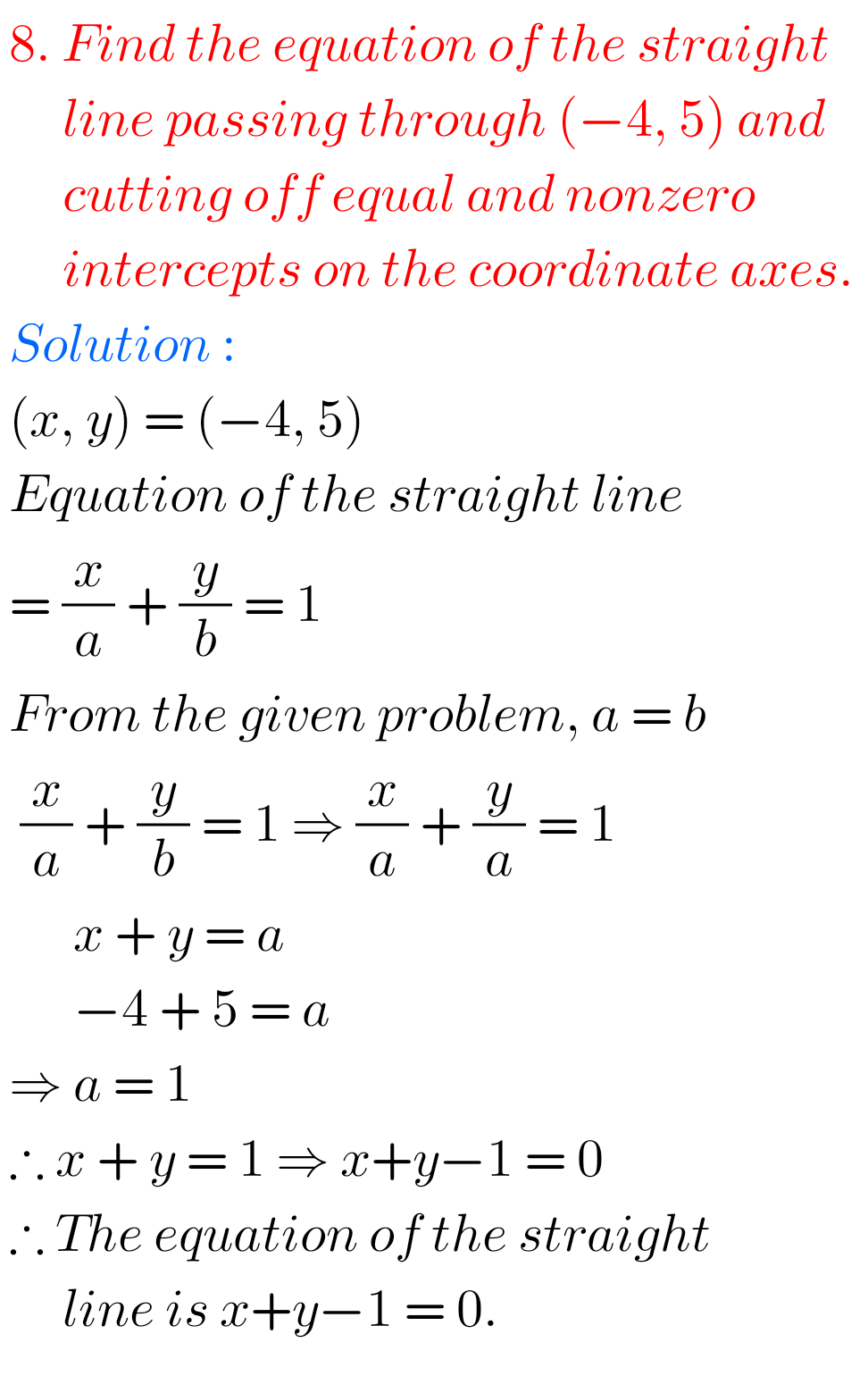## Inter maths solutions for The Straight Line Exercise 3 (a)

Intermediate Maths Solutions for The Straight Line Exercise 3(a) The Straight Line Exercise 3(a) Maths 1b textbook Chapter 3 Inter Solutions are given. All problems with answers are given. These are very simple to understand. Observe the example problems and solutions in the textbook. Observe the given solutions and try them in your own method. …